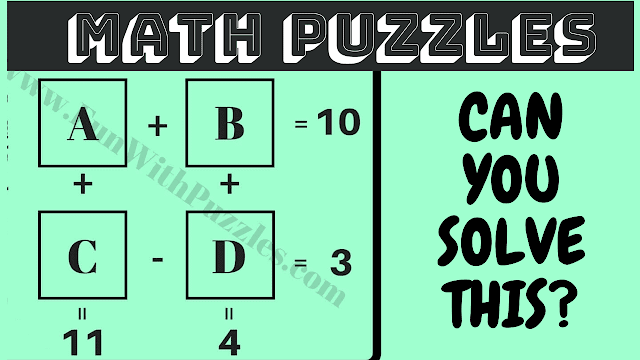This Puzzle Video contains the mathematical code-breaking puzzle questions which will twist your mind. In this puzzle video, there are 5 puzzle images. Each puzzle contains the maths puzzle question in which there are some number equations. Your challenge in these puzzles is to break the mathematical codes and find the values of A, B, C, and D.
You will get 15 seconds to solve each of these code-breaking puzzles. However, if you feel that 15 seconds is very little to solve any of these puzzles, you can pause the video and then solve these puzzle questions. I am sure that you will be able to solve most of these maths puzzles. Write down your answer to the last bonus puzzle in the comments.